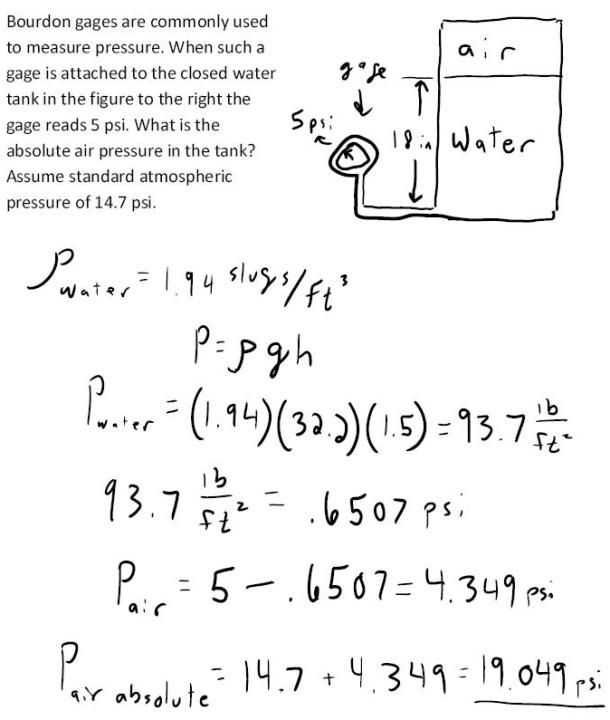fluid mechanics math problems engineering equations fluid mechanics basic mechanics school homework engineering math fluids formulas fluid mechanics problems fluid mechanics problem solutions to fluids problems full solution fluid mechanics engineering fluids problem solution fluid mechanics math problems engineering equations fluid mechanics basic mechanics school homework engineering math fluid mechanics formulas fluid problems fluid mechanics problem solutions to fluid mechanics problems full solution fluid mechanics engineering fluid mechanics problem solution
fluid mechanics math problems engineering equations fluid mechanics basic mechanics school homework engineering math fluids formulas fluid mechanics problems fluid mechanics problem solutions to fluids problems full solution fluid mechanics engineering fluids problem solution fluid mechanics math problems engineering equations fluid mechanics basic mechanics school homework engineering math fluid mechanics formulas fluid problems fluid mechanics problem solutions to fluid mechanics problems full solution fluid mechanics engineering fluid mechanics problem solution
Highalphabet Home Page fluid mechanics solutions fluids math solved Fluid Mechanics Page 1
Bourdon gages are commonly used to measure pressure. When such a gage is attached to the closed water tank in the figure to the right the gage reads 5 psi. What is the absolute air pressure in the tank? Assume standard atmospheric pressure of 14.7 psi.Bourdon gages are commonly used to measure pressure. When such a gage is attached to the closed water tank in the figure to the right the gage reads 5 psi. What is the absolute air pressure in the tank? Assume standard atmospheric pressure of 14.7 psi.